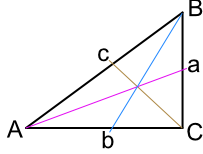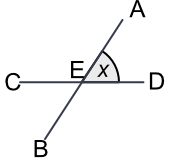Labelling Conventions

## Labelling Conventions

Angles and triangles are labelled in a standard manner.

An angle is named with three letters, in capitals.On the diagram above, the angle at A is labelled as BAC, with the angle letter in the middle.

Triangles are labelled with three pairs of letters: a, b and c and A, B and C. The lower case letters, a, b and c represent the lengths, and the upper case letters are the angles.

For each pair of letters, the length letters are opposite the angle letters.

For right-angled triangles, label the right angle as C (to make sense of Pythagoras theorem a2 + b2 = c2).On a right-angled triangle, the longest side is known as the hypotenuse.

## Example 1

In a right-angled triangle, the right angle is labelled C. What is the hypotenuse labelled?

The hypotenuse is the name for the longest side in a right-angled triangle, and is opposite the right angle (which should be labelled as C).